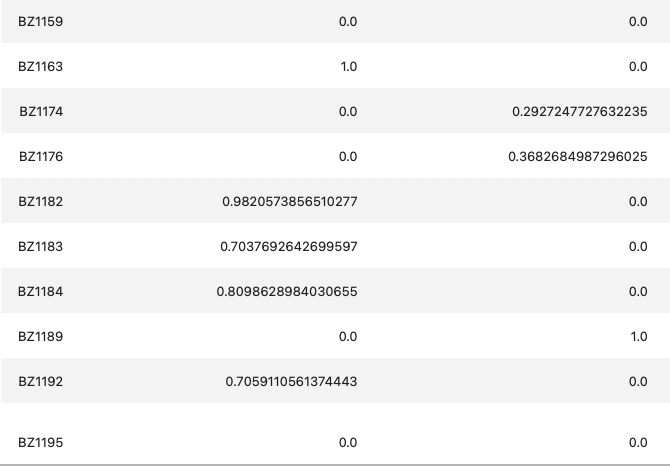# taxonomy barplot csv file table

Hello, I have a question about qiime taxonomy barplot.
When you open the csv file after creating a taxonomy barplot, the sum of the percentage of features per sample is not 1.

What's the reason?
Finally, the bar plot is drawn to fill up 100 percent, but why doesn't the sum come up to 1 in numbers?

If you take the table in the csv file and convert it back to the absolute value for each sample, it changes to a smaller number than the original value..Shouldn't it be converted so that the total is 1? I wonder if there is a reason why it appears like this in qiime.

(For your information, I used feature-classify classify-consensus-blast for taxonomy assignment.)

Hello!

That's right - in that table numbers are the summary of all ASVs counts, that were assigned to certain taxa in that sample. Plugin will convert them to percentages for figures, but csv tables are not converted. If you want to get percentage or ratios, you will need to divide numbers by the total count in a sample and (optionally for %) multiply it by 100.

I have some difficulties to follow this part of the question. Please, feel free to ask it here in this thread again if my answer does not explain the issue.

Best,
Timur

1 Like

Hello @timanix ! thank you for your kind apply

So, according to you, the number of the table itself does not seem to be changed to the absolute value, but the bar plot being drawn is drawn as a converted number, so the total comes out to 100? I don't know if I understand correctly.

The reason why I had this question is that when I drew a barplot using a module like matplotlib in Pandas without using the qiime plug-in, it came out a little different from the figure in the table used to draw the barplot.

This is qiime taxonomy barplot output csv fileThis is the value that I changed from Pandas to absolute value (it is a percentage of the number of features divided by the number of features added to each sample)

As you can see it makes a little difference. Generally, the value I processed separately is larger than the qiime output result. The resulting bar plot also had a sample that showed a slight difference (such as a slight difference in the size of the bar,
I wonder which value is more reliable in this case and why qiime handles it in this way.

I'm sorry for the long question. Thank you.

Hello again,

Are we talking about the same thing? In the CSV table from taxonomy barplot visualization absolute counts are given. It is why they do not sum to 1 or 100 (in that case they should be relative frequencies or abundances).

Yes, in the visualization itself relative abundances are given.

I had the same issue when drawing barplots in pandas / matplotlib, and the differences were arising from:

1. Filtering. For example, if you used some filtering threshold in Qiime2 before taxabarplot creation, and then in Pandas you took unfiltered counts, then there should be some differences.
2. Order of actions. For example, in Qiime2 counts first collapsed by taxa and then converted to relative frequencies. If you changed the order, it can also affect the output.

Is it after converting table to relative abundances outside of qiime2?
In qiime2 csv table from taxabarplots, counts are absolute frequencies and >= 0, while in your example they are < 0.

You mean, after converting absolute counts to relative abundances?
All this "1.0" looks very suspicious to me. Is it 1%? Exactly 1.0% in so many samples? Or 1.0 means 100%?
Are the number of columns (features, taxa) is the same in 2 tables?
When I convert absolute counts to relative (%), I use this formula in pandas:

`df = df.div(df.sum(axis = 1), axis = 0) * 100`

Just skip "* 100" if you want relative frequencies instead of percentage.

This topic was automatically closed 31 days after the last reply. New replies are no longer allowed.